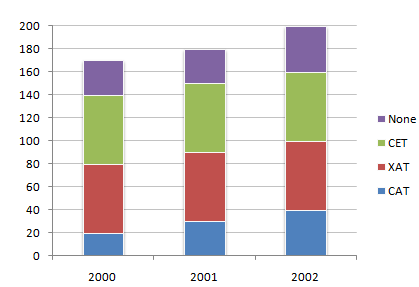# Data Interpretation - Bar Charts - Discussion

Discussion Forum : Bar Charts - Bar Chart 14 (Q.No. 3)
Directions to Solve

The following chart represents the number of students who passed the CAT exam or the XAT exam or the CET exam or None of these exams. (Assume that there are no students who passed more than one exam.)

Number of students who qualified CAT/XAT/CET Exams3.
What is the percentage increase in the number of students in 2002 over 2000 ?
30 %
17.64 %
117.6 %
85 %
Explanation:
Total percentage increase in the number of students in 2002 over 2000 is = (30 x 100)/170 = 17.64 %
Discussion:
2 comments Page 1 of 1.

Vijayalaxmi said:   8 years ago
Total percentage increase in the number of students in 2002 over 2000 is:

= ((200-170)/170)*100.
= (30/170)*100.

= 3000/170.
= 17.64.

Priya said:   10 years ago
How 30 gets?